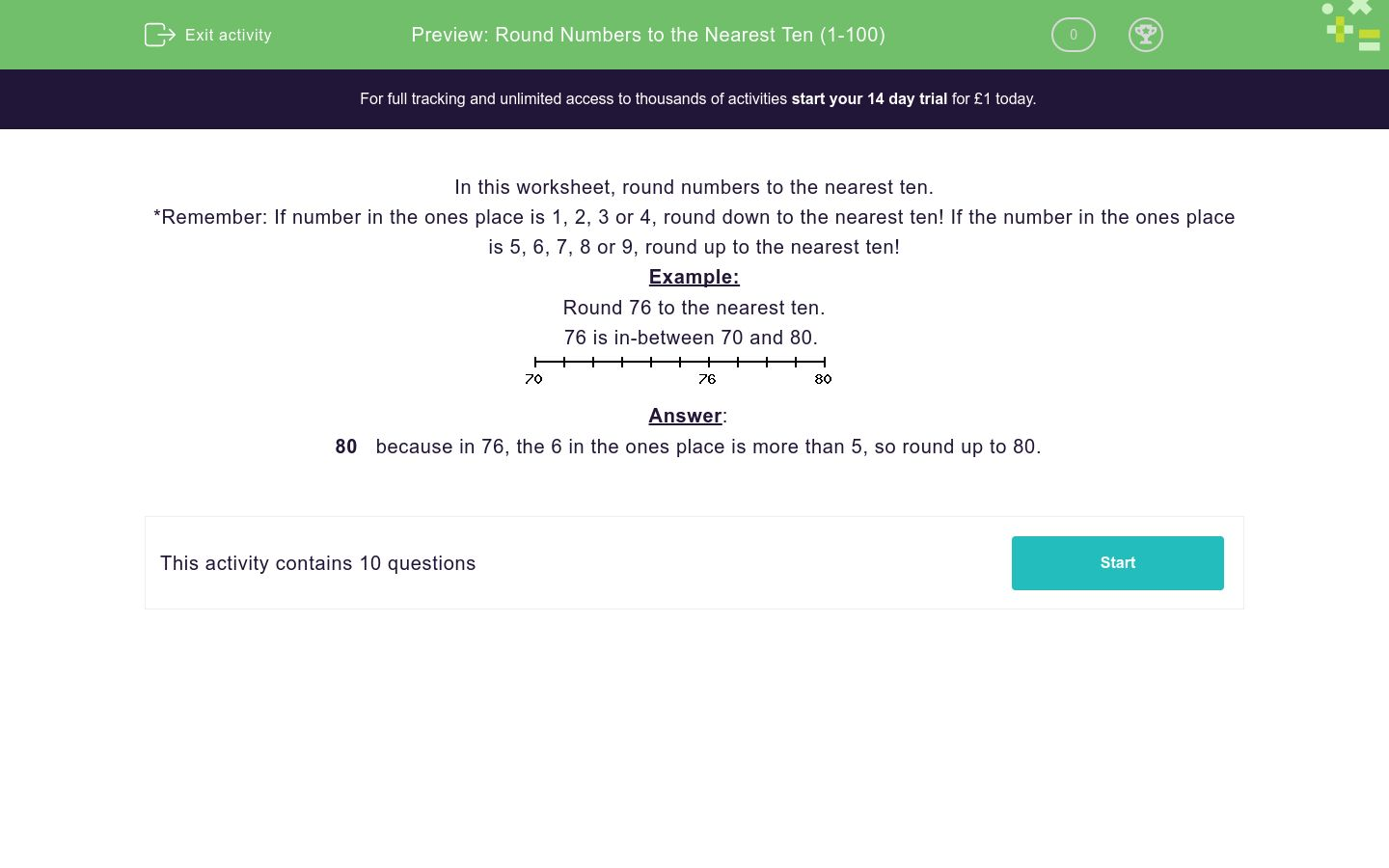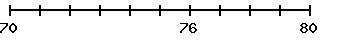# Round Numbers to the Nearest Ten (1-100)

In this worksheet, students round numbers to the nearest ten.Key stage:  KS 2

Curriculum topic:   Number: Number and Place Value

Curriculum subtopic:   Round Numbers Up/Down (to 10, 100 or 1000)

Difficulty level:### QUESTION 1 of 10

In this worksheet, round numbers to the nearest ten.

*Remember: If number in the ones place is 1, 2, 3 or 4, round down to the nearest ten! If the number in the ones place is 5, 6, 7, 8 or 9, round up to the nearest ten!

Example:

Round 76 to the nearest ten.

76 is in-between 70 and 80.80   because in 76, the 6 in the ones place is more than 5, so round up to 80.

Round 82 to the nearest ten.

Round 88 to the nearest ten.

Round 55 to the nearest ten.

Round 36 to the nearest ten.

Round 17 to the nearest ten.

Round 59 to the nearest ten.

Round 13 to the nearest ten.

Round 44 to the nearest ten.

Round 53 to the nearest ten.

Round 23 to the nearest ten.

• Question 1

Round 82 to the nearest ten.

80
EDDIE SAYS
82 is in-between 80 and 90. The 2 in the ones place is less than 5, so round down to 80.
• Question 2

Round 88 to the nearest ten.

90
EDDIE SAYS
88 is in-between 80 and 90. The 8 in the ones place is greater than 5, so round up to 90.
• Question 3

Round 55 to the nearest ten.

60
EDDIE SAYS
55 is in-between 50 and 60. There is a 5 in the ones place, so round up to 60.
• Question 4

Round 36 to the nearest ten.

40
EDDIE SAYS
36 is in-between 30 and 40. The 6 in the ones place is greater than 5, so round up to 40.
• Question 5

Round 17 to the nearest ten.

20
EDDIE SAYS
17 is in-between 10 and 20. The 7 in the ones place is more than 5, so round up to 20.
• Question 6

Round 59 to the nearest ten.

60
EDDIE SAYS
59 is in-between 50 and 60. The 9 in the ones place is greater than 5, so round up to 60.
• Question 7

Round 13 to the nearest ten.

10
EDDIE SAYS
13 is in-between 10 and 20. The 3 in the ones place is less than 5, so round down to 10.
• Question 8

Round 44 to the nearest ten.

40
EDDIE SAYS
44 is in-between 40 and 50. The 4 in the ones place is less than 5, so round down to 40.
• Question 9

Round 53 to the nearest ten.

50
EDDIE SAYS
53 is in-between 50 and 60. The 3 in the ones place is less than 5, so round down to 50.
• Question 10

Round 23 to the nearest ten.

20
EDDIE SAYS
23 is in-between 20 and 30. The 3 in the ones place is less than 5, so round down to 20.
---- OR ----

Sign up for a £1 trial so you can track and measure your child's progress on this activity.

### What is EdPlace?

We're your National Curriculum aligned online education content provider helping each child succeed in English, maths and science from year 1 to GCSE. With an EdPlace account you’ll be able to track and measure progress, helping each child achieve their best. We build confidence and attainment by personalising each child’s learning at a level that suits them.

Get started